# Extract or Filter Top n values

You might have been through this kind of situation where you need to filter out the top n values from the list having few values, and I am also pretty sure about it that you might have chosen to do it manually, which is also a great choice when you have only a few values in a list, and you want to filter out the top n values.

But if you are dealing with multiple values in the list and you want to filter out the top n values, then in such a situation doing these tasks manually would be a foolish act because by doing it manually, there are 90% chances that you would 100% get tired of it and can’t complete your task at the right time.

But don’t be worry about it because after carefully reading this article filtering out the top n values from the list containing multiple values would become a piece of cake for you.

So let’s dive into the article to take you out of this fix.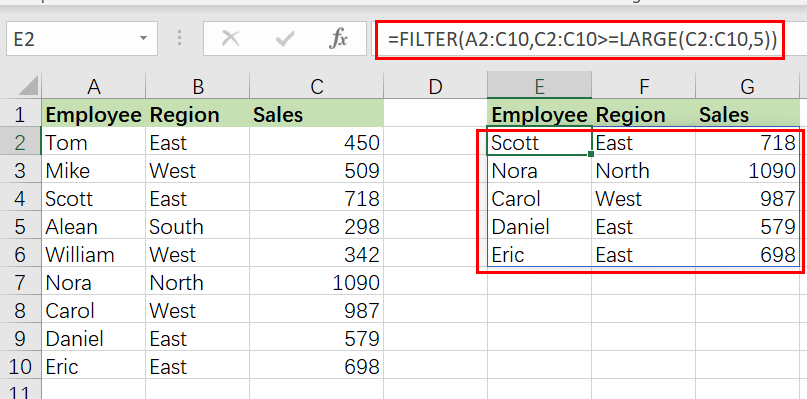## General Formula

The Following formula would help you Filter on top values in MS Excel:

`=FILTER(input_range,condition_range>=LARGE(condition_range,n))`## Syntax Explanations

Before going into the explanation of the formula for getting the work done efficiently, we must understand each syntax which would make it easy for us that how each syntax contributes to filtering out the top n values in MS Excel.

• `Filter`: This function contributes to narrowing down or filtering out a range of data on the user-defined criteria.
• `input_range`: In your worksheet, it represents the input ranges.
• `Comma symbol` (,): In Excel, this comma symbol acts as a separator that helps to separate a list of values.
• `condition_range`: In Excel, the range is nothing but the difference between highest and lowest values
• `Parenthesis` (): The core purpose of this Parenthesis symbol is to group the elements and separate them from the rest of the elements.
• `Greater than Equal To Symbol` (>=): Greater than Equal To Symbol (>=) is used to identify the values which are either greater than or equal to.
• `LARGE``:` Large function is used to get the higher arguments.

## Let’s See How This Formula Works

For instance, you got a task in which there is a table where you have candidates of two groups (i.e., group A and group B ) and which are assigned to a particular sales value, now you want to filter out the top 5 candidates with the higher sales, now let’s analyze that how to to write the formula and how this formula would do it.

As to filter on the top n values, we would write the formula according to the given list like:

`=FILTER(TotalData,Sales>=LARGE(Sales,5))`

The FILTER function is used in this formula to obtain data based on a logical test built using the LARGE function.

The LARGE function is a straightforward method for obtaining the nth largest number in a range. Specify a range as the first parameter  and an n value as the second argument:

`=LARGE(Sales,5)// Fifth largest`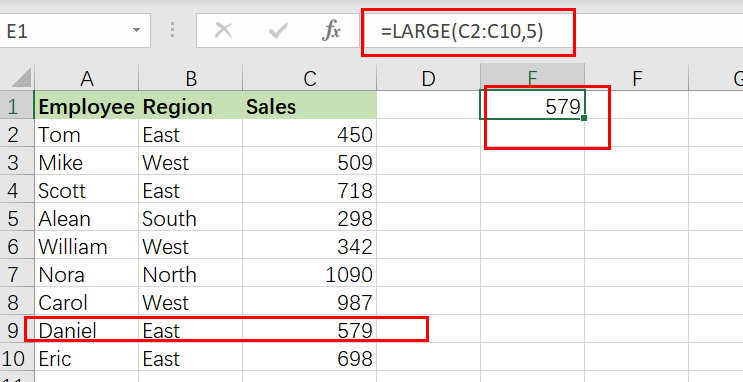This snippet is used to build the logic for FILTER in this example:

`=Sales>=LARGE(Sales,5)`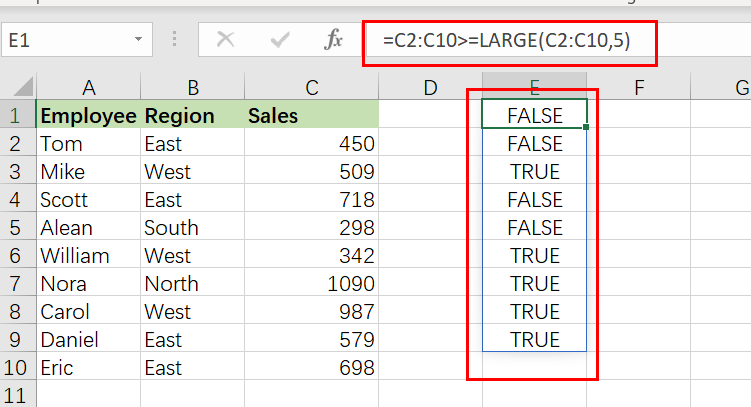Moreover, the above snippet would return `TRUE` if the sales is larger than or equal to the fifth-highest sales. Because there are 9 cells in the range A2:A10, this equation yields an array of 9 TRUE and FALSE values in the form of the following array:

`{FALSE;FALSE;TRUE;FALSE;FALSE;TRUE;TRUE; TRUE;FALSE}`

This array is returned as the included parameter to the FILTER function, which filters the data. Only rows with the result TRUE are included in the final output.

The algorithm in `E2` produces the highest five sales in the total data, which fall into the range` E2:G6`.

## Sort results by Sales Value Using Sort Function

FILTER will return matched entries in the order they occur in the supplied data by default.

If you want to sort results by the sales result in decreasing order, you just need to nest the original FILTER formula within the SORT function as follows:

`=SORT(FILTER(TotalData,Sales>=LARGE(Sales,5)),3,-1)`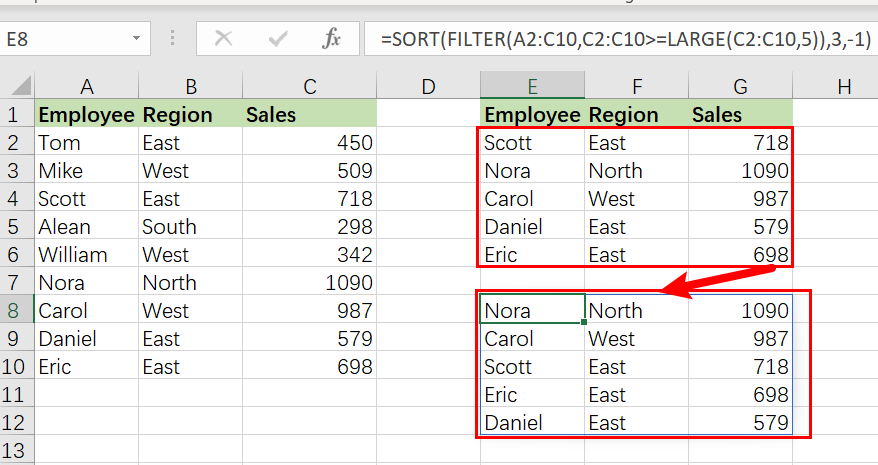In this case, FILTER passes the results to the SORT function as an array parameter. The sort index is set to 3(sort results based on Sales values),while the sort order is set to -1 (descending order).

## Extract Top N Values Using XLOOKUP formula

Users of Microsoft 365 may achieve the same effects by utilizing the new XLOOKUP function:

`=XLOOKUP(LARGE(\$B\$2:\$B\$12, D3), \$B\$2:\$B\$12, \$A\$2:\$A\$12), \$B\$2:\$A\$12)`

In this scenario, LARGE returns the kth largest number as the search value straight to XLOOKUP.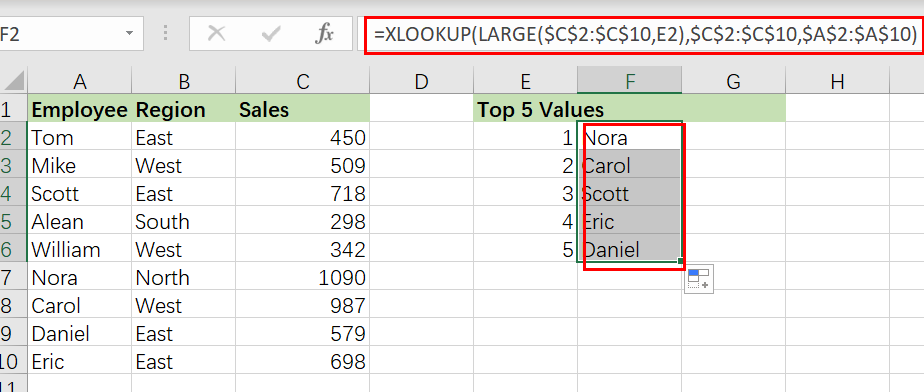Using the XLOOKUP formula, you may find matches to the top or bottom values.

This syntax is significantly more accessible than the INDEX MATCH formula. However, please remember that XLOOKUP is only accessible in Excel 365. This formula will not function with Excel 2019, Excel 2016, or older versions.

### Related Functions

• Excel LARGE function
The Excel LARGE function returns the largest numeric value from the numbers that you provided. Or returns the largest value in the array. The syntax of the LARGE function is as below:= LARGE (array,nth)…
• Excel INDEX function
The Excel INDEX function returns a value from a table based on the index (row number and column number)The INDEX function is a build-in function in Microsoft Excel and it is categorized as a Lookup and Reference Function.The syntax of the INDEX function is as below:= INDEX (array, row_num,[column_num])…
• Excel MATCH  function
The Excel MATCH function search a value in an array and returns the position of that item.The MATCH function is a build-in function in Microsoft Excel and it is categorized as a Lookup and Reference Function.The syntax of the MATCH function is as below:= MATCH  (lookup_value, lookup_array, [match_type])….
• Excel Filter function
The FILTER function extracts matched records from a collection of data using one or more logical checks. The include argument specifies logical tests, which might encompass a wide variety of formula conditions.==FILTER(array,include,[if empty])…
• Excel Sort function
The SORT function in Excel sorts the contents of an array or range alphabetically or numerically by columns or rows.The syntax:=SORT(array, [sort index],) …